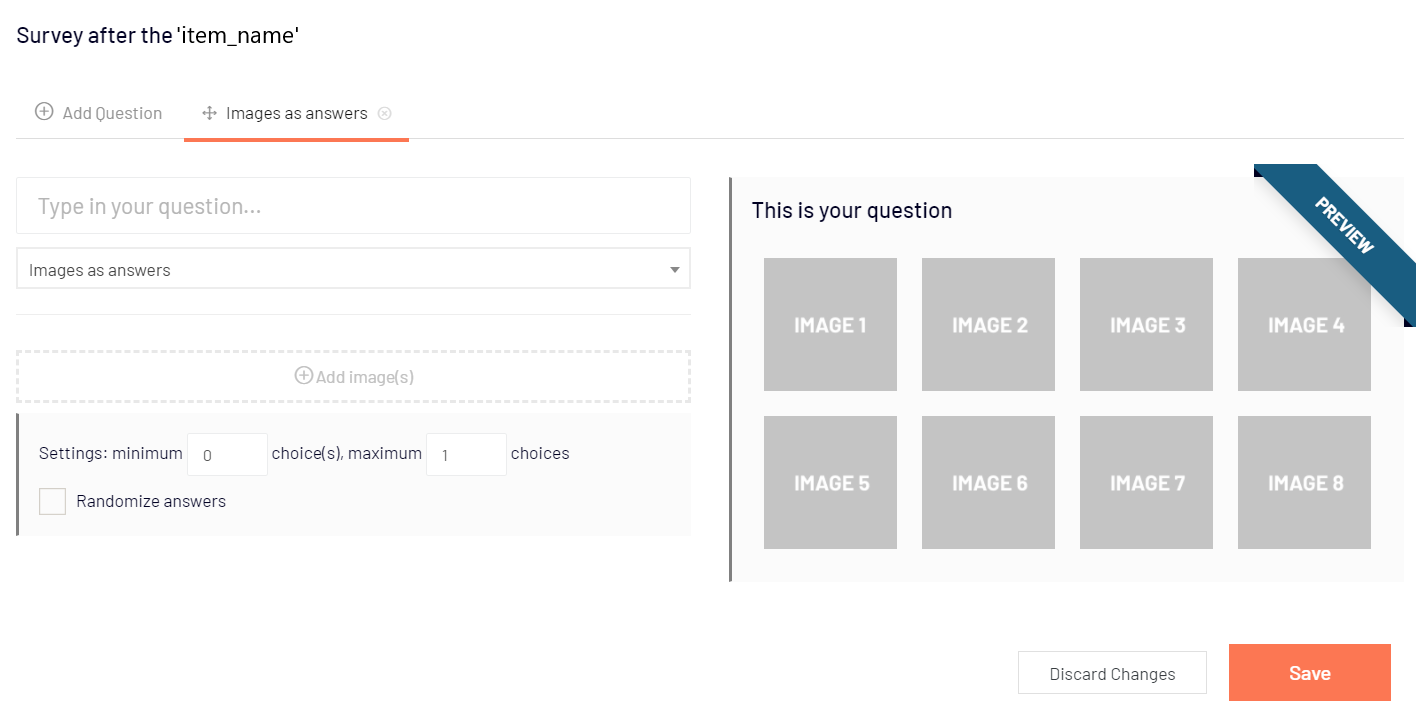# Survey module

After each stimulus (either image, video or embedded website), you can add several follow-up questions to obtain additional insights for your research. For website mockups and screen recording studies, you can create a survey at the end of the study.

You can add the following types of questions: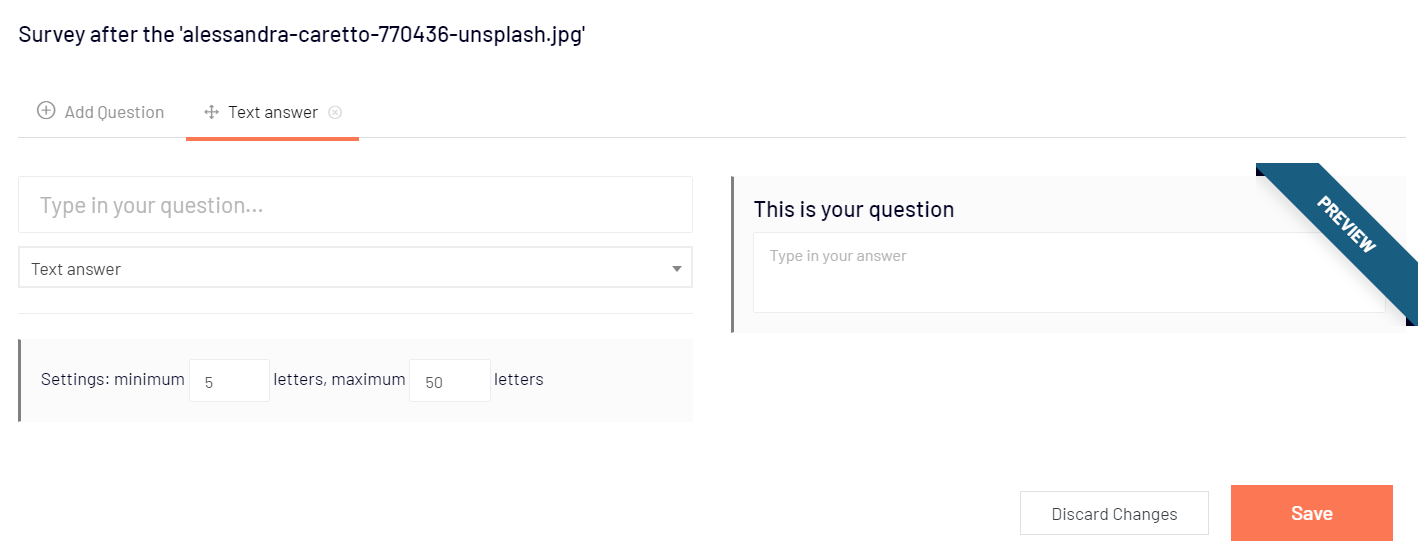This is a question where a participant has to type the answer manually - you can set the minimum and the maximum number of characters required. If the minimum number of letters is set to 0, then answering the question isn't required.

Example: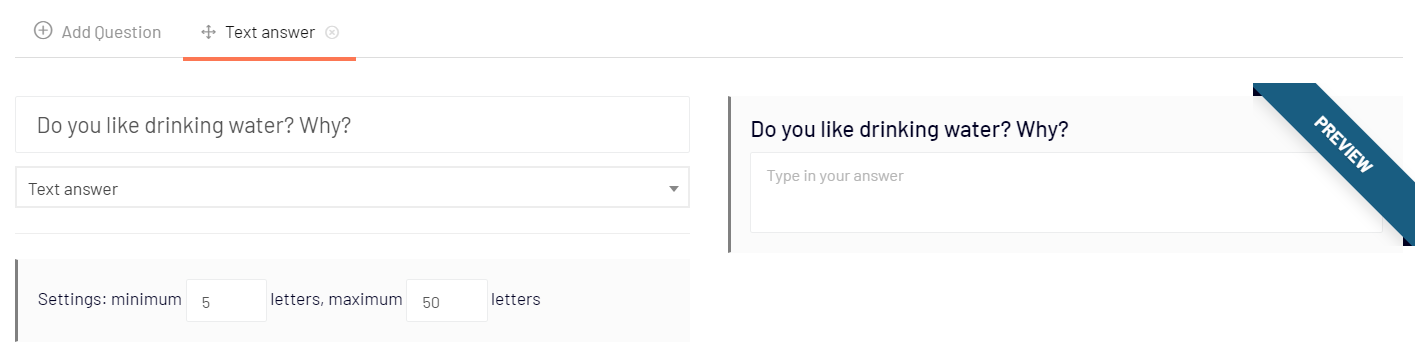How it looks for participants: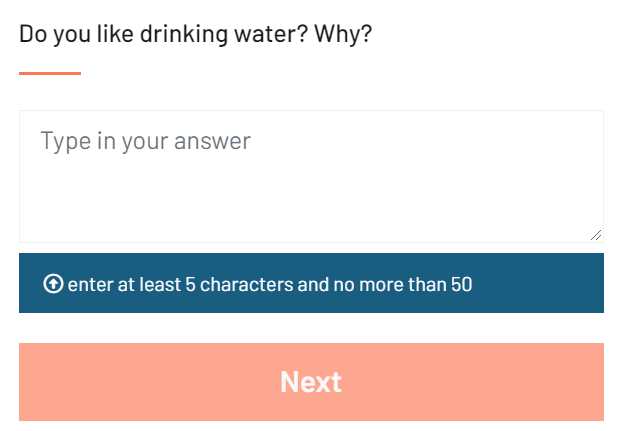• choice question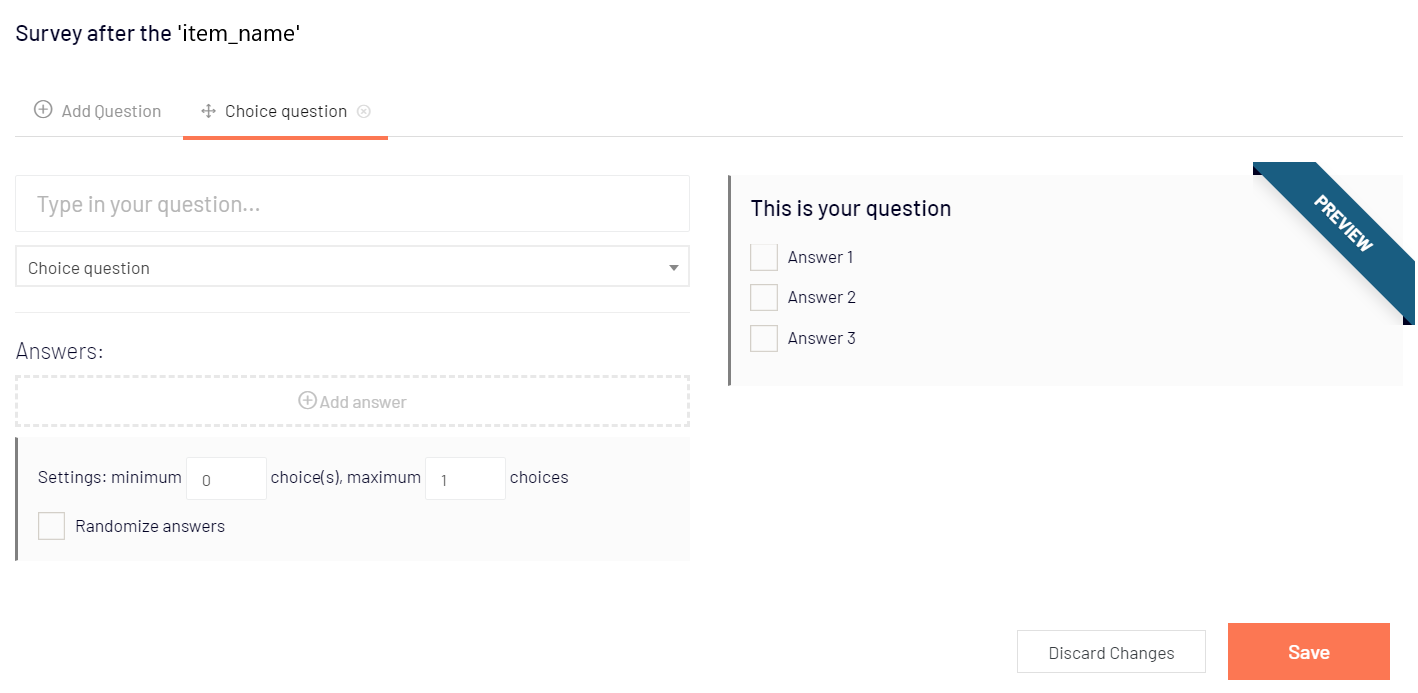For this type of question, you give a participant some options to choose from (they can be displayed in random order if the "Randomize answers" option is checked). You can set how many answers can be chosen - if the minimum number of choices is 0, then the answer to this question is not required. To make participants choose exactly 1 answer, set the minimum and the maximum number of choices allowed to 1.

Example: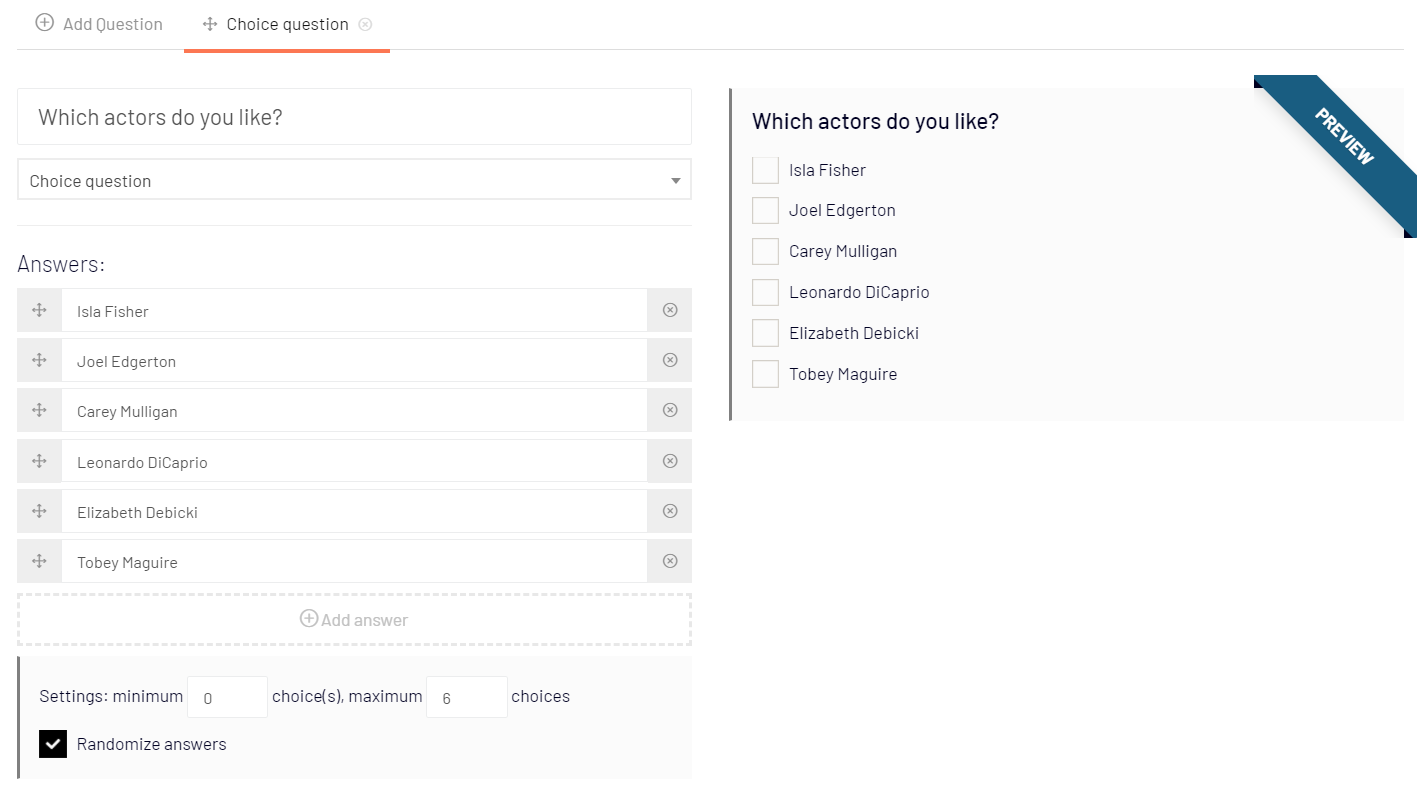How it looks for participants: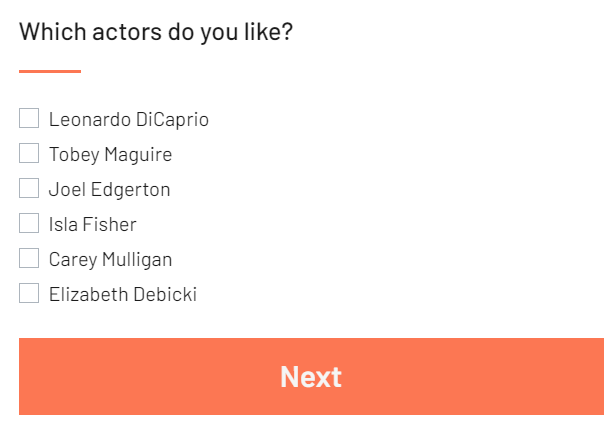In this case, the minimum number of choices was set to 0, so a participant can skip the question without choosing any of the actors - the "Next" button is active without any option checked.

• 1-5 rating scale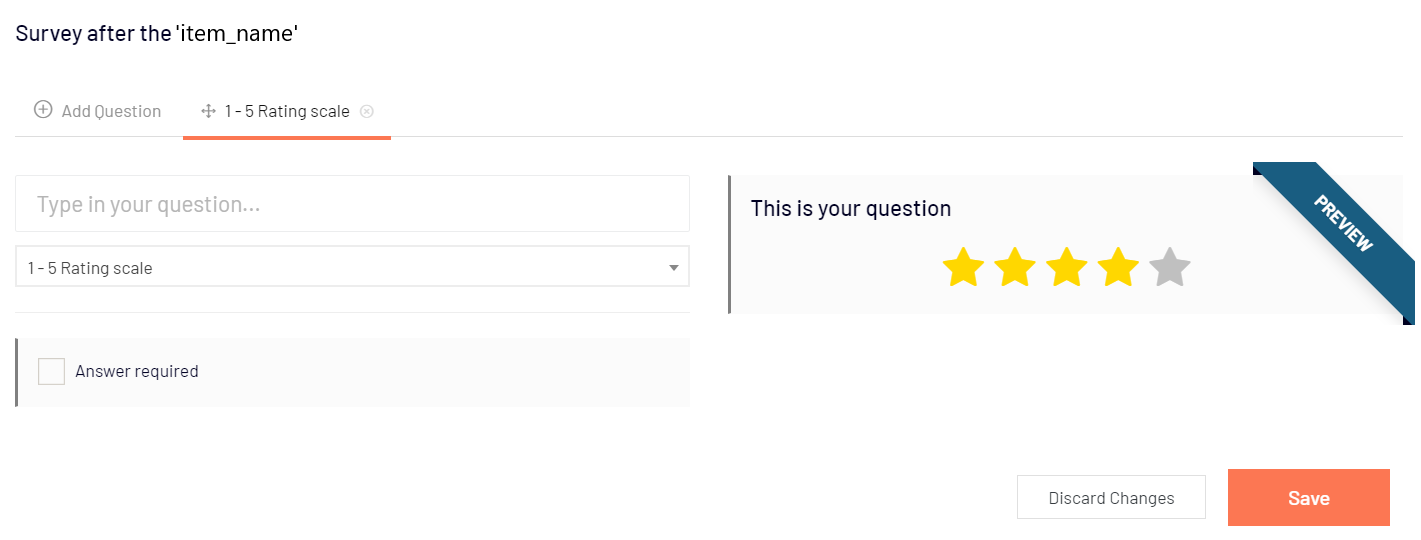Ask participants to rate something (i.e., an ad that they have just seen) on a scale from 1 to 5. You may (or may not) set an answer to this question as required.

Example: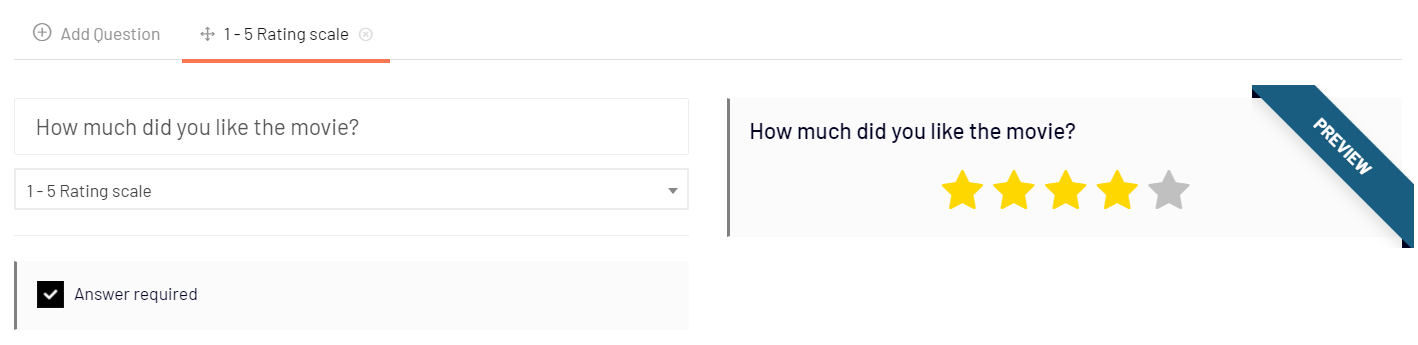How it looks for participants: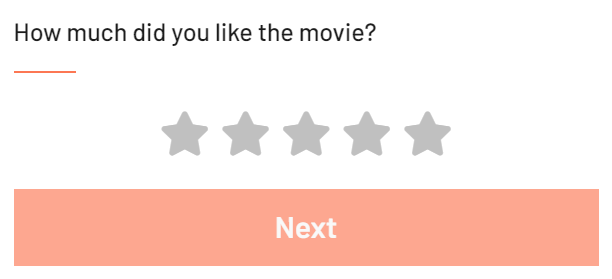The answer, in this case, was required as the "Next" button remains inactive.

• matrix choice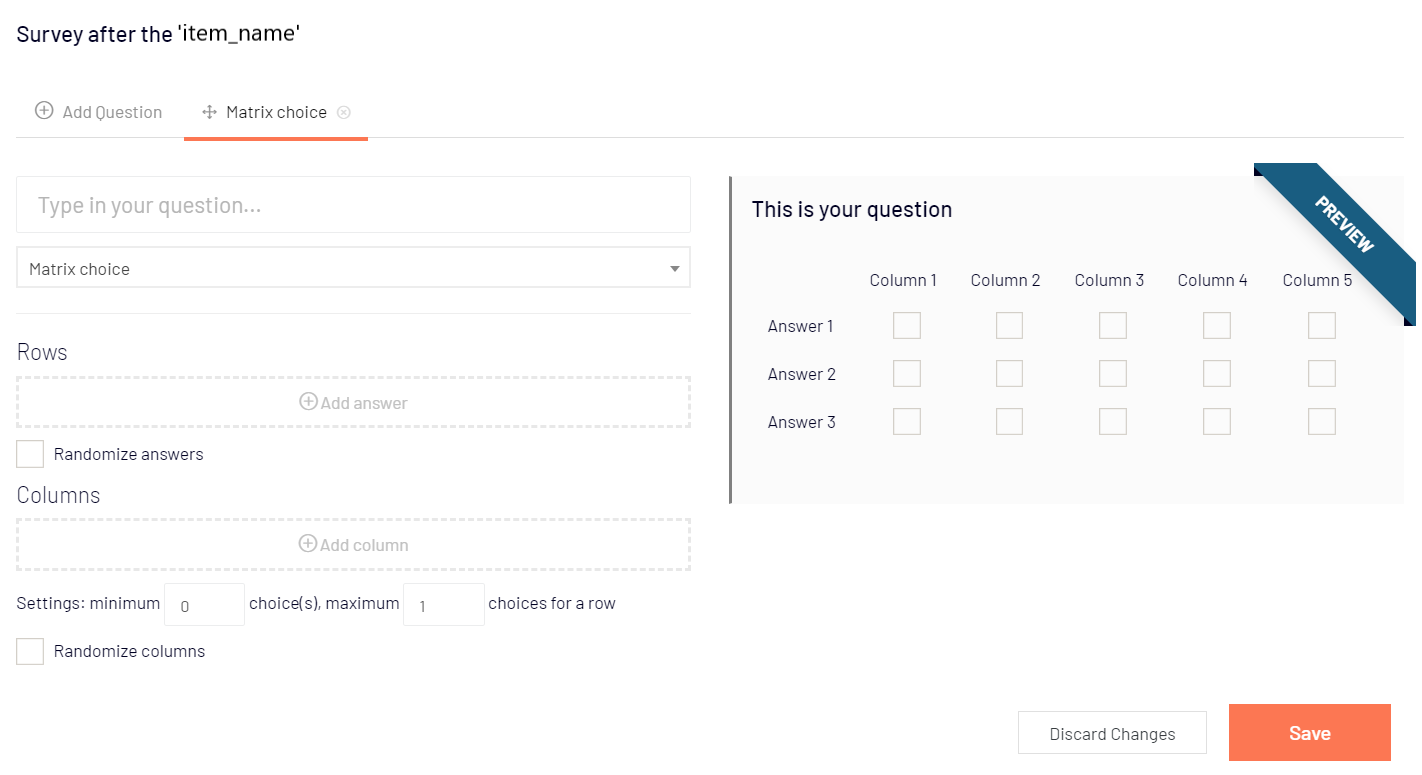A matrix choice is a type of question that consists of a group of multiple-choice (or single-choice) questions displayed as a grid of rows and columns. For example, if you put in rows actors and in columns traits, you can ask the participant to assign to each actor some of the traits, that best describe them (multiple-choice). Another example, this time for single-choice answers, would be to rate products (in rows) by checking the right column (with grades).

You can randomize the order of rows, columns, or both and choose how many choices (from columns) can be chosen for each row (including 0 - no answer required or any exact value if the minimum and the maximum number of choices are set to the same value).

Example: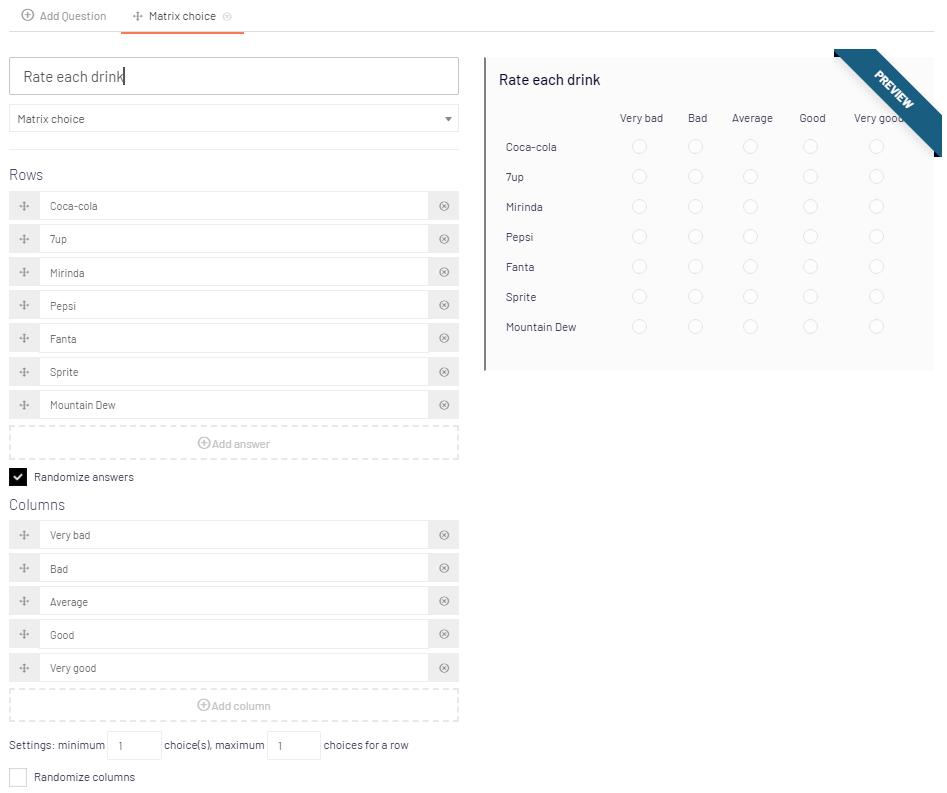How it looks for participants: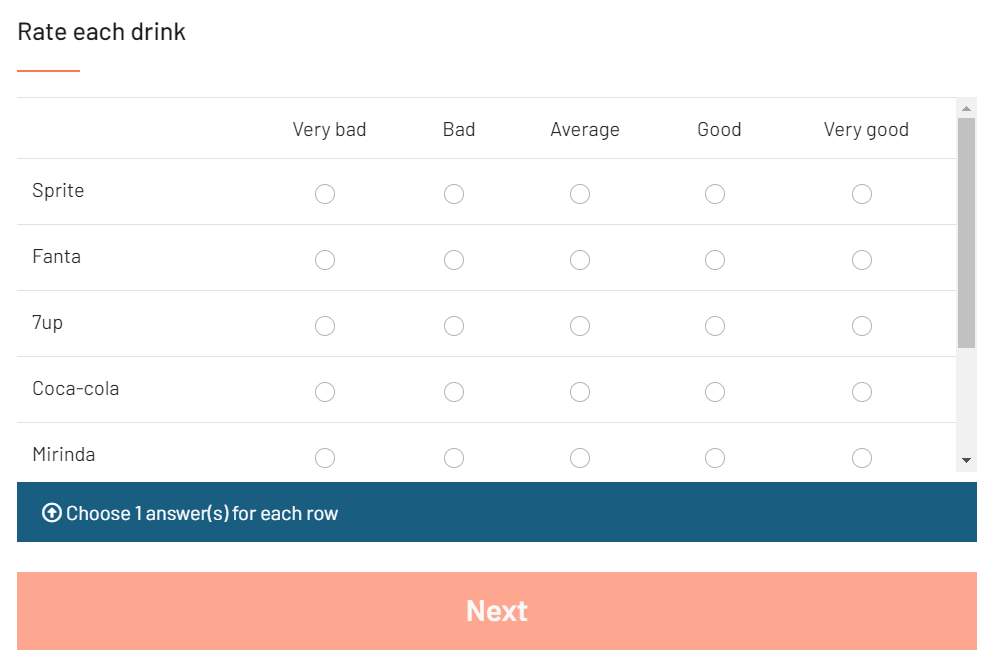For each drink, exactly 1 answer (column) could be chosen.